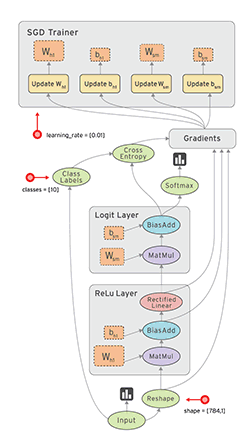# 背景## tensorflow 特点

• 高度的灵活性

TensorFlow 不是一个严格的“神经网络”库。只要你可以将你的计算表示为一个数据流图，你就可以使用Tensorflow。你来构建图，描写驱动计算的内部循环。

• 真正的可移植性

Tensorflow 在CPU和GPU上运行，比如说可以运行在台式机、服务器、手机移动设备等等。

• 自动求微分

基于梯度的机器学习算法会受益于Tensorflow自动求微分的能力。作为Tensorflow用户，你只需要定义预测模型的结构，将这个结构和目标函数（objective function）结合在一起，并添加数据，Tensorflow将自动为你计算相关的微分导数。

• 多语言支持

Tensorflow 有一个合理的c++使用界面，也有一个易用的python使用界面来构建和执行你的graphs。你可以直接写python/c++程序。Go，Java，Lua，Javascript，或者是R 的API也在不断的开发中。

• 性能最优化

比如说你又一个32个CPU内核、4个GPU显卡的工作站，想要将你工作站的计算潜能全发挥出来？由于Tensorflow 给予了线程、队列、异步操作等以最佳的支持，Tensorflow 让你可以将你手边硬件的计算潜能全部发挥出来。你可以自由地将Tensorflow图中的计算元素分配到不同设备上，Tensorflow可以帮你管理好这些不同副本。

# tensorflow上手使用

## 使用tensorflow用平面拟合三维数据

#coding=utf-8
'''

'''
import tensorflow as tf
import numpy as np

# 使用 NumPy 生成假数据(phony data), 总共 100 个点.
x_data = np.float32(np.random.rand(2, 100)) # 随机输入
y_data = np.dot([0.100, 0.200], x_data) + 0.300

# 构造一个线性模型
b = tf.Variable(tf.zeros())
W = tf.Variable(tf.random_uniform([1, 2], -1.0, 1.0))
y = tf.matmul(W, x_data) + b

# 最小化方差
loss = tf.reduce_mean(tf.square(y - y_data))
train = optimizer.minimize(loss)

# 初始化变量
init = tf.initialize_all_variables()

# 启动图 (graph)
sess = tf.Session()
sess.run(init)

# 拟合平面
for step in xrange(0, 201):
sess.run(train)
if step % 20 == 0:
print step, sess.run(W), sess.run(b)
# 得到最佳拟合结果 W: [[0.100 0.200]], b: [0.300]

[root@master tensorflow]# python demo2.py
I tensorflow/core/common_runtime/local_device.cc:25] Local device intra op parallelism threads: 24
I tensorflow/core/common_runtime/local_session.cc:45] Local session inter op parallelism threads: 24
0 [[-0.49180511  0.69824928]] [ 0.76379919]
20 [[-0.0375709   0.23476422]] [ 0.35483342]
40 [[ 0.0724101   0.20012772]] [ 0.31455269]
60 [[ 0.09411168  0.1987025 ]] [ 0.30379418]
80 [[ 0.09867454  0.19946699]] [ 0.30097923]
100 [[ 0.09968913  0.19983345]] [ 0.30025125]
120 [[ 0.09992492  0.1999529 ]] [ 0.30006427]
140 [[ 0.09998151  0.19998731]] [ 0.30001637]
160 [[ 0.09999539  0.19999665]] [ 0.30000418]
180 [[ 0.09999884  0.19999914]] [ 0.30000106]
200 [[ 0.09999971  0.19999979]] [ 0.30000025]

## tensorflow要点

• 使用图 (graph) 来表示计算任务.
• 在被称之为 会话 (Session) 的上下文 (context) 中执行图.
• 使用 tensor 表示数据.
• 通过 变量 (Variable) 维护状态.
• 使用 feed 和 fetch 可以为任意的操作(arbitrary operation) 赋值或者从其中获取数据.

## tensorflow使用

1. 定义tensorflow变量

b = tf.Variable(tf.zeros())
W = tf.Variable(tf.random_uniform([1, 2], -1.0, 1.0))

2. 定义运算

y = tf.matmul(W, x_data) + b

3. 定义损失函数，优化函数，优化目标

loss = tf.reduce_mean(tf.square(y - y_data))
train = optimizer.minimize(loss)

4. 准备初始化所有变量

init = tf.initialize_all_variables()

5. 启动图会话，初始化所有变量

sess = tf.Session()
sess.run(init)

6. 迭代更新参数到好最优解

for step in xrange(0, 201):
sess.run(train)